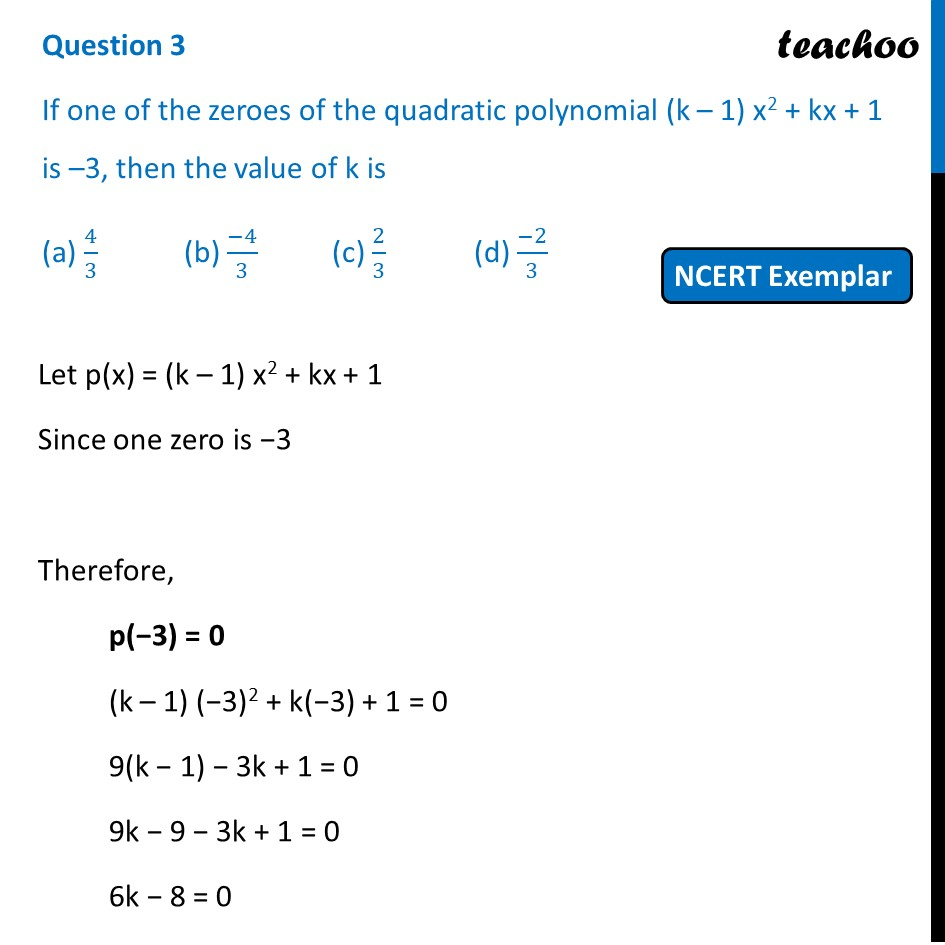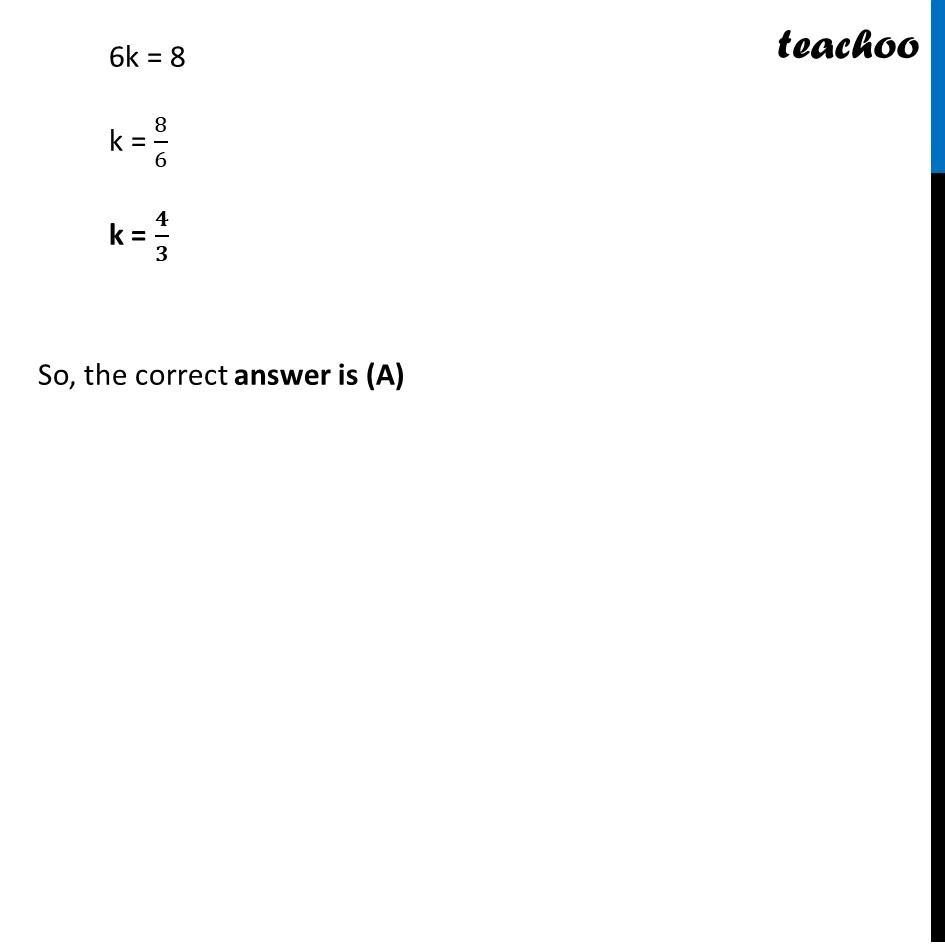## (a) 4/3       (b) (-4)/3  (c) 2/3    (d) (-2)/31. Chapter 2 Class 10 Polynomials (Term 1)
2. Serial order wise
3. MCQs from NCERT Exemplar

Transcript

Question 3 If one of the zeroes of the quadratic polynomial (k – 1) x2 + kx + 1 is –3, then the value of k is (a) 4/3 (b) (−4)/3 (c) 2/3 (d) (−2)/3 Let p(x) = (k – 1) x2 + kx + 1 Since one zero is −3 Therefore, p(−3) = 0 (k – 1) (−3)2 + k(−3) + 1 = 0 9(k − 1) − 3k + 1 = 0 9k − 9 − 3k + 1 = 0 6k − 8 = 0 6k = 8 k = 8/6 k = 𝟒/𝟑 So, the correct answer is (A)

MCQs from NCERT Exemplar